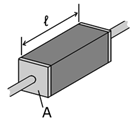# Material Libraries

From the menu bar, click Properties > Material Library. The Materials dialog opens. Built in and user defined material library can be managed using this menu. Use the Materials dialog to add, modify, and remove certain materials and export the materials data as Material File (*.mtrl) which could be used by import function.

By double-clicking a certain material in the Materials dialog or selecting and then clicking Edit, the material properties can be modified. Clicking Add Dielectric or Add Conductor opens the Edit dialog with empty values.

For conducting material, user should define the electric resistivity, relative magnetic permeability and thermal conductivity along to x, y and z axis. For dielectric material, dielectric constant, loss tangent and thermal conductivity along to x, y and z axis would be needed.

You can save and re-load material file by clicking Import and Export.

The short descriptions for the representative properties of dielectric and conductive materials are in table below.

Properties Description
Conductivity Electrical conductivity or specific conductance is a measure of a material's ability to conduct an electric current. When an electrical potential difference is placed across a conductor, its movable charges flow, giving rise to an electric current. The conductivity σ is defined as the ratio of the current density $J$ to the electric field strength $E$ :
$J=\sigma E$
It is also possible to have materials in which the conductivity is anisotropic, in which case σ is a 3×3 matrix (or more technically a rank-2 tensor), which is generally symmetric. Conductivity is the reciprocal (inverse) of electrical resistivity, ρ, and has the SI units of siemens per meter (S·m-1) and CGSE units of inverse second (s–1):
$\sigma =\frac{1}{p}$
Resistivity Electrical resistivity ρ (Greek: rho) is defined by,
$p=\frac{E}{J}$
Where $p$ is the static resistivity (measured in volt-meters per ampere, V m/A); $E$ is the magnitude of the electric field (measured in volts per meter, V/m); $J$ is the magnitude of the current density (measured in amperes per square meter, A/m2). The electrical resistivity $p$ can also be given by,$p=R\frac{A}{\mathcal{l}}$
Where $p$ is the static resistivity (measured in ohm-meters, Ω m); $R$ is the electrical resistance of a uniform specimen of the material (measured in ohms, Ω); $\mathcal{l}$ is the length of the piece of material (measured in meters, m); $A$ is the cross-sectional area of the specimen (measured in square meters, m²). Finally, electrical resistivity is also defined as the inverse of the conductivity σ (sigma), of the material, or
$p=\frac{1}{\sigma }$
Magnetic Permeability In electromagnetism, permeability is the measure of the ability of a material to support the formation of a magnetic field within itself. In other words, it is the degree of magnetization that a material obtains in response to an applied magnetic field. Magnetic permeability is typically represented by the Greek letter $\mu$ . The reciprocal of magnetic permeability is magnetic reluctivity.

In SI units, permeability is measured in the henry per meter (H m-1), or newton per ampere squared (N A-2). The permeability constant ( ${\mu }_{0}$ , also known as the magnetic constant or the permeability of free space, is a measure of the amount of resistance encountered when forming a magnetic field in a classical vacuum. The magnetic constant has the exact value ${\mu }_{0}$ = 4π×10−7 ≈ 1.2566370614...×10−6 H·m-1 or N·A-2).

Thermal Conductivity Thermal conductivity is the quantity of heat transmitted through a unit thickness in a direction normal to a surface of unit area, due to a unit temperature gradient under steady state conditions. Thermal conductivity is same term with heat transfer coefficients. 1 W/(mK) = 1 W/(moC) = 0.85984 kcal/(hr moC) = 0.5779 Btu/(ft hr oF)
Dielectric Constant A dielectric is an electrical insulator that may be polarized by the action of an applied electric field. When a dielectric is placed in an electric field, electric charges do not flow through the material, as in a conductor, but only slightly shift from their average equilibrium positions causing dielectric polarization. Because of dielectric polarization, positive charges are displaced along the field and negative charges shift in the opposite direction. This creates an internal electric field which partly compensates the external field inside the dielectric. If a dielectric is composed of weakly bonded molecules, those molecules not only become polarized, but also reorient so that their symmetry axis aligns to the field.

While the term insulator refers to a low degree of electrical conduction, the term "dielectric" is typically used to describe materials with a high polarizability. The latter is expressed by a number called the dielectric constant. A common, yet notable, example of a dielectric is the electrically insulating material between the metallic plates of a capacitor. The polarization of the dielectric by the applied electric field increases the capacitor's capacitance.

Loss Tangent The loss tangent is a parameter of a dielectric material that quantifies its inherent dissipation of electromagnetic energy. The term refers to the angle in a complex plane between the resistive (lossy) component of an electromagnetic field and its reactive (lossless) component.